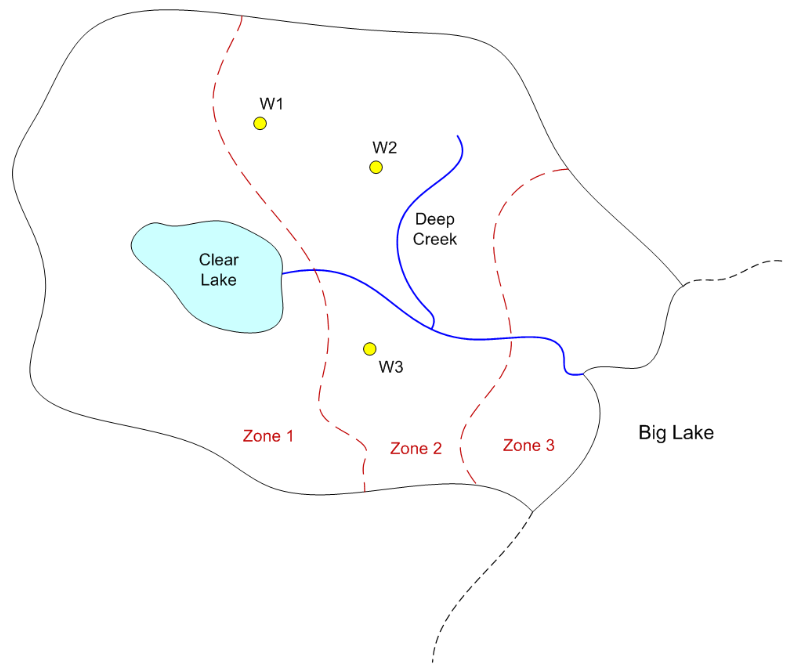# MODFLOW Conceptual Model Approach - Big Lake

For this exercise we will create a MODFLOW model for the following site using the conceptual model approach:To build the model, do the following:

1) Download and unzip the following file. Launch GMS and open the project file (start.gpr) contained in the zip archive.

biglake.zip

2) Set units (m, d)

3) Create a MODFLOW conceptual model

4) Create a boundary coverage using three arcs. Put two separate arcs on right side next to the lake.

5) Duplicate the boundary coverage to make a source/sink coverage. Turn on the following attributes:

Wells
Refine points
River

6) Use the Build Polygon command to build a variable head polygon that defines the active area.

7) Create spec head boundary on Big Lake side

8) Create river arcs

a) Calculate conductance per unit length

width = 5 m

vertical thickness = 2 m

K = 0.01 m/d

Cond = Kw/t = (0.01)(5)/(2) = 0.025

b) Assign heads and bottom elevations

Head at lake = 120, Rbot = 117

Head at upper right end = 118, Rbot = 115

Head at junction = 110, Rbot = 107

Head at bottom = 100, Rbot = 97

9) Create general head boundary for lake. Build polygons.

a) Stage = 120 m

b) Calculate conductance per unit area

Vertical thickness = 4 m

K = 0.01 m/d

Cond = K/t = 0.01/4 =  0.0025

10) Create the wells

a) Refine Data (for all)

Base size = 10

Bias = 1.1

Max cell size = 40

b) Flowrates

Q1 = -4000 m^3/d

Q2 = -3000

Q3 = -2500

11) Duplicate the boundary coverage to make a K coverage. Build three polygons corresponding to the three zones shown on the map. Enter the following K values:

Zone 1: K= 1

Zone 2: K = 2

Zone 3: K = 0.5

12) Duplicate the boundary coverage to build a recharge-elev coverage. Turn on the recharge, top elevation, and bottom elevation attributes. Build a single polgyon and assign the following values:

Recharge = 0.0002 m/d

Top = 120 m

Bottom = 10 m

13) Create a grid frame and fit it to the site.

14) Build the grid.

15) Initialize MODFLOW

16) Activate cells in coverage

17) Map -> MODFLOW

18) Run the Model Checker

19) Save and run the model